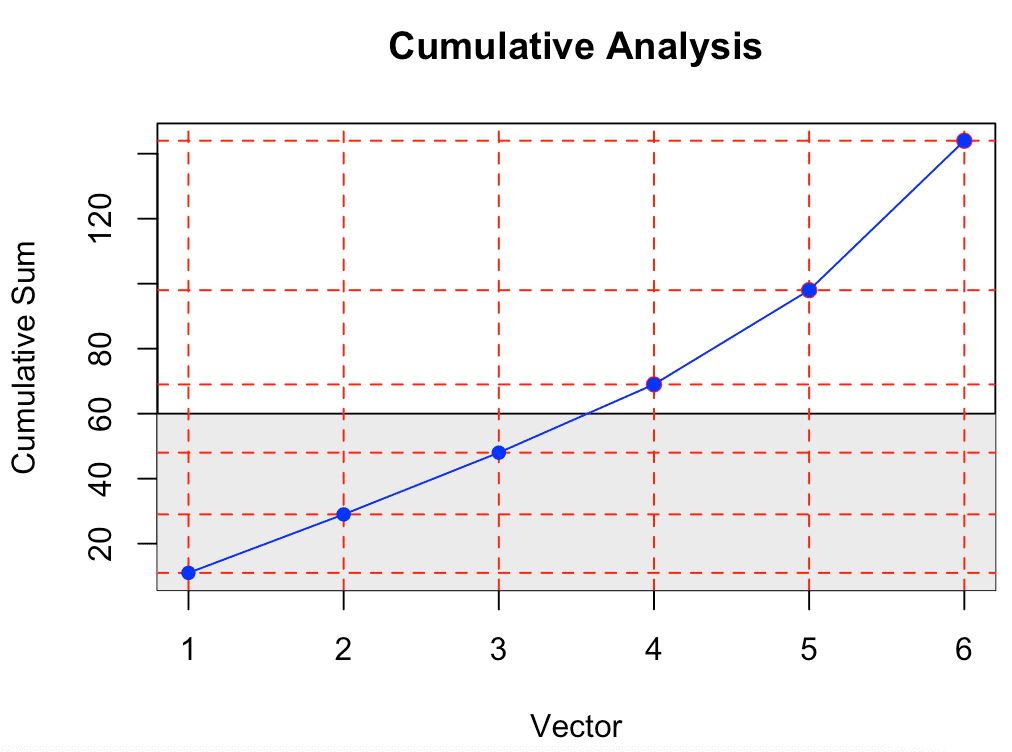# cumsum in R: Calculate Cumulative Sum of a Numeric Object

In data processing, the cumulative sum of the specific data objects is an exciting part. You need to calculate the sum of all values up to a particular position of data. In this example, you will see how to calculate the cumulative sum of a numerical object.

## cumsum in R

The cumsum() is a built-in R function that calculates the cumulative sum of the vector. The cumsum() function returns a vector whose elements are the cumulative sums of the elements passed as an argument.

### Syntax

``cumsum(x)``

### Parameters

It takes a Numeric Object as an argument.

### Return value

It returns a vector of the same length and type as a passed argument.

## Using cumsum() function on Vector

To create a vector in R, use the colon(:) operator. Let’s create two vectors and pass that to the cumsum() function.

``````cumsum(1:5)
cumsum(-1:-5)``````

#### Output

`````` 1 3 6 10 15
 -1 -3 -6 -10 -15``````

You can see that the output vector is filled with the cumulative sum of the 1:5 values. Internally, it calculates like this:

1,

1+2 = 3,

1+2+3 = 6,

1+2+3+4 = 10,

1+2+3+4+5 = 15

and hence we got 1, 3, 6, 10, 15. This is the same for the negative values in which we got -1, -3, -6, -10, -15.

Let’s create a vector consists of float values.

``````dt <- c(1.1, 1.9, 2.1)
dt2 <- c(1.8, 2.1, 4.6)

cumsum(dt)
cumsum(dt2)``````

#### Output

`````` 1.1 3.0 5.1
 1.8 3.9 8.5``````

## How to Plot a Graph of cumsum() function in R

To plot a graph of cumsum() function in R, use the plot() function. The plot() function provides a nice way to visualize the cumulative sum output. Let’s take the following vector as data.

``data <- c(11, 18, 19, 21, 29, 46)``

To find the cumulative sum of the Vector in R, use the cumsum() function.

``````data <- c(11, 18, 19, 21, 29, 46)
cmsm <- cumsum(data)
cmsm``````

#### Output

`` 11 29 48 69 98 144``

Now, let’s plot this output on a cumulative chart to analyze the data quickly.

``````data <- c(11, 18, 19, 21, 29, 46)
cmsm <- cumsum(data)

plot(
x = seq_len(length(cmsm)),
y = cmsm,
col = "red",
xlab = "Vector",
ylab = "Cumulative Sum", main = "Cumulative Analysis"
)

rect(0, 60, 11, 0,
border = "black",
col = "grey92"
)

abline(
v = seq_len(length(cmsm)),
col = "red",
lty = "dashed"
)

abline(
h = cmsm,
col = "red",
lty = "dashed"
)

points(
x = seq_len(length(cmsm)),
y = cmsm,
col = "blue",
type = "l"
)

points(
x = seq_len(length(cmsm)),
y = cmsm,
col = "blue",
pch = 16
)``````

Now, run this file in RStudio, and you will see the following cumulative chart.## Applying cumsum() function to a Real Data Frame

To create a data frame in R, use the data.frame() function. Let’s create a numeric data frame.

``````data <- data.frame(
x1 = c(11, 21, 19, 46),
x2 = c(51, 15, 11, 14),
x3 = c(19, 21, 13, 41)
)``````

Let’s apply the cumsum() method to this data frame. We will pass columns one by one.

``````data <- data.frame(
x1 = c(11, 21, 19, 46),
x2 = c(51, 15, 11, 14),
x3 = c(19, 21, 13, 41)
)

cumsum(data\$x1)
cumsum(data\$x2)
cumsum(data\$x3)``````

#### Output

`````` 11 32 51 97
 51 66 77 91
 19 40 53 94``````

## How to deal with missing values in cumsum() function

In a large dataset, there are always missing values to deal with. Missing values need to be addressed while using the cumsum() method; otherwise, it returns NA.

``````data <- c(11, 18, 19, 21)
data_na <- data
data_na[c(3, 4)] <- NA
cumsum(data_na)
``````

#### Output

`` 11 29 NA NA``

In this example, we assign index 3 and 4 to NA and then pass that vector to the cumsum() function, and hence it returns the NA in the output, which is not feasible output.

Unfortunately, the na.rm option is not available within the cumsum() function. However, you can use the is.na() method that provides a good option.

``````data <- c(11, 18, 19, 21)
data_na <- data
data_na[c(3, 4)] <- NA
cumsum(data_na[!is.na(data_na)])``````

#### Output

`` 11 29``

You can see that we have ignored all the NA values, and it returns the correct output without any NA values.

That’s it for the cumsum() function in R.

sum in r

colSums in r

rowSums in r

round in r

min in r

max in r

Categories R